# Build Map and Localize Using Segment Matching

This example shows how to build a map with lidar data and localize the position of a vehicle on the map using SegMatch , a place recognition algorithm based on segment matching.

Autonomous driving systems use localization to determine the position of the vehicle within a mapped environment. Autonomous navigation requires accurate localization, which relies on an accurate map. Building an accurate map of large scale environments is difficult because the map accumulates drift over time, and detecting loop closures to correct for accumulated drift is challenging due to dynamic obstacles. The SegMatch algorithm is robust to dynamic obstacles and reliable in large scale environments. The algorithm is a segment-based approach that takes advantage of descriptive shapes and recognizes places by matching segments.

### Overview

Like the Build a Map from Lidar Data Using SLAM example, this example uses 3-D lidar data to build a map and corrects for the accumulated drift using graph SLAM. However, this example does not require global pose estimates from other sensors, such as an inertial measurement unit (IMU). After building the map, this example uses it to localize the vehicle in a known environment.

In this example, you learn how to:

• Use SegMatch to find the relative transformation between two point clouds that correspond to the same place

• Build a map using SegMatch for loop closure detection

• Localize on a prebuilt map using SegMatch

The data used in this example is part of the Velodyne SLAM Dataset. It includes approximately 6 minutes of data recorded with a Velodyne® HDL64E-S2 scanner. Download the data to a temporary directory. This can take a few minutes.

```baseDownloadURL = 'https://www.mrt.kit.edu/z/publ/download/velodyneslam/data/scenario1.zip'; dataFolder = fullfile(tempdir, 'kit_velodyneslam_data_scenario1', filesep); options = weboptions('Timeout', Inf); zipFileName = dataFolder + "scenario1.zip"; % Get the full file path to the PNG files in the scenario1 folder. pointCloudFilePattern = fullfile(dataFolder, 'scenario1', 'scan*.png'); numExpectedFiles = 2513; folderExists = exist(dataFolder, 'dir'); if ~folderExists % Create a folder in a temporary directory to save the downloaded zip file. mkdir(dataFolder) disp('Downloading scenario1.zip (153 MB) ...') websave(zipFileName, baseDownloadURL, options); % Unzip downloaded file. unzip(zipFileName, dataFolder) elseif folderExists && numel(dir(pointCloudFilePattern)) < numExpectedFiles % Redownload the data if it got reduced in the temporary directory. disp('Downloading scenario1.zip (153 MB) ...') websave(zipFileName, baseDownloadURL, options); % Unzip downloaded file. unzip(zipFileName, dataFolder) end```
```Downloading scenario1.zip (153 MB) ... ```

The downloaded dataset stores point cloud data in PNG files. Create a file datastore using the `helperReadVelodyneSLAMData` function to load point cloud data from the PNG files and convert distance values to 3-D coordinates. The helper function is a custom read function, which is designed for the Velodyne SLAM Dataset. Select a subset of the data and split the data to use for map building and for localization.

```% Create a file datastore to read files in the right order. fileDS = fileDatastore(pointCloudFilePattern, 'ReadFcn', ... @helperReadVelodyneSLAMData); % Read the point clouds. ptCloudArr = readall(fileDS); % Select a subset of point cloud scans, and split the data to use for % map building and for localization. ptCloudMap = vertcat(ptCloudArr{1:5:1550}); ptCloudLoc = vertcat(ptCloudArr{2:5:1550}); % Visualize the first point cloud. figure pcshow(ptCloudMap(1)) title('Point Cloud Data')```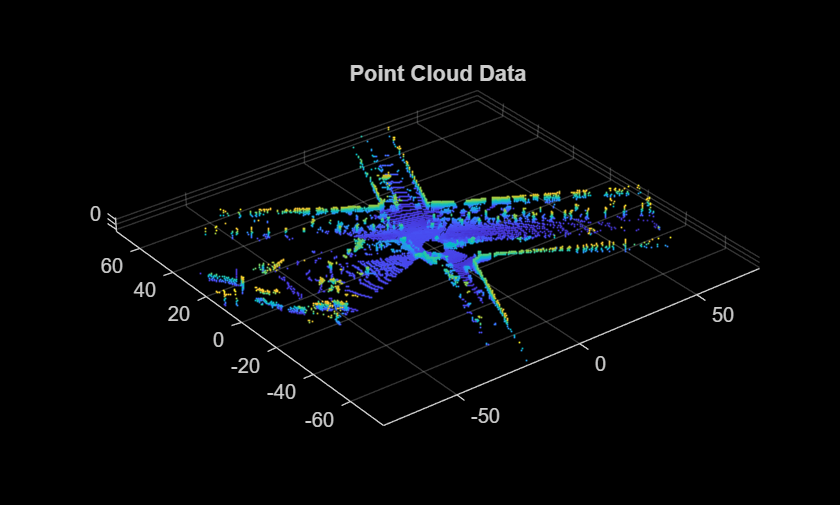### SegMatch Overview

The SegMatch algorithm consists of four different components: point cloud segmentation, feature extraction, segment matching, and geometric verification. For best results, preprocess the point cloud before performing these four steps.

#### Preprocess Point Cloud

To select the most relevant point cloud data, perform the following preprocessing steps:

1. Select a cylindrical neighborhood centered around the vehicle to extract a local point cloud of interest. First, specify a cylindrical neighborhood based on the distance of the points from the origin in the x and y directions. Then, select the area of interest using `select`.

2. Remove the ground in preparation to segment the point cloud into distinct objects. Use `segmentGroundSMRF` (Lidar Toolbox) to segment the ground.

```% Select a point cloud from the map for preprocessing. ptCloud = ptCloudMap(25); % Set the cylinder radius and ego radius. cylinderRadius = 40; egoRadius = 1; % Compute the distance between each point and the origin. dists = hypot(ptCloud.Location(:,:,1), ptCloud.Location(:,:,2)); % Select the points inside the cylinder radius and outside the ego radius. cylinderIdx = dists <= cylinderRadius & dists >= egoRadius; cylinderPtCloud = select(ptCloud, cylinderIdx, 'OutputSize', 'full'); % Remove the ground. [~, ptCloudNoGround] = segmentGroundSMRF(cylinderPtCloud, 'ElevationThreshold', 0.05); % Visualize the point cloud before and after preprocessing. figure pcshowpair(ptCloud, ptCloudNoGround) title('Point Cloud Before and After Preprocessing')```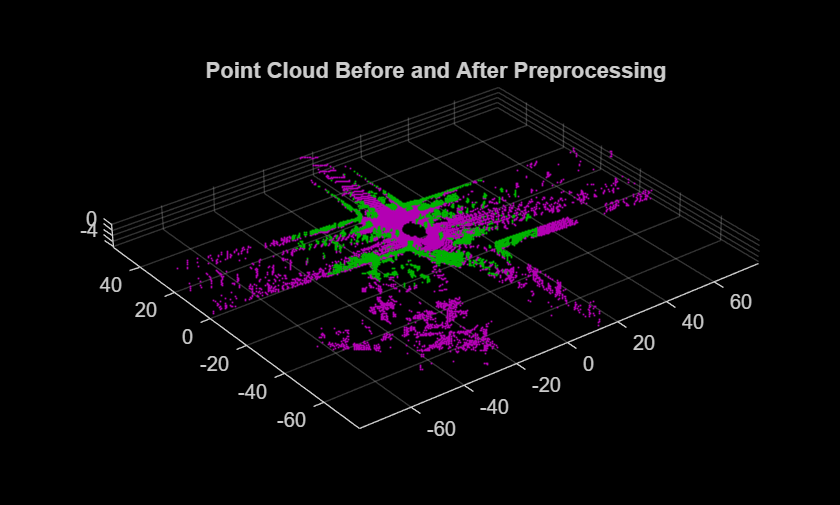#### Segmentation and Feature Extraction

Next, segment the point cloud and extract features from each segment.

Segment the point cloud by using the `segmentLidarData` function and visualize the segments. For this example, each segment must have a minimum of 150 points. This produces segment clusters that represent distinct objects and have enough points to characterize the area in the map.

Different datasets require different parameters for segmentation. Requiring fewer points for segments can lead to false positive loop closures, and limiting the segments to larger clusters can eliminate segments that are important for place recognition. You must also tune the distance and angle thresholds to ensure that each segment corresponds to one object. A small distance threshold can result in many segments that correspond to the same object, and a large distance threshold and small angle threshold can result in segments that combine many objects.

```minNumPoints = 150; distThreshold = 1; angleThreshold = 180; [labels, numClusters] = segmentLidarData(ptCloudNoGround, distThreshold, ... angleThreshold, 'NumClusterPoints', minNumPoints); % Remove points that contain a label value of 0 for visualization. idxValidPoints = find(labels); labelColorIndex = labels(idxValidPoints); segmentedPtCloud = select(ptCloudNoGround, idxValidPoints); figure pcshow(segmentedPtCloud.Location, labelColorIndex) title('Point Cloud Segments')```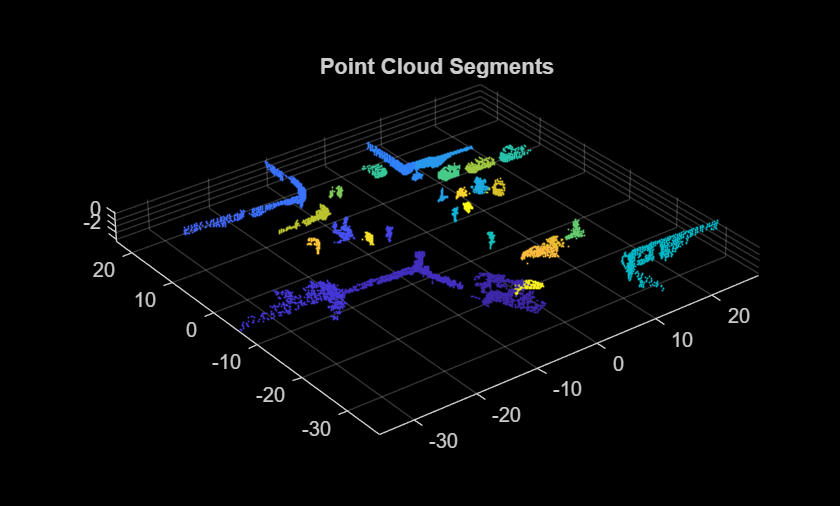Extract features from each segment by using the `extractEigenFeatures` (Lidar Toolbox) function. Eigenvalue-based features are geometric features. Each feature vector includes linearity, planarity, scattering, omnivariance, anisotropy, eigenentropy, and change in curvature.

```[features, segments] = extractEigenFeatures(ptCloud, labels); disp(features)```
``` 31×1 eigenFeature array with properties: Feature Centroid ```
`disp(segments)`
``` 31×1 pointCloud array with properties: Location Count XLimits YLimits ZLimits Color Normal Intensity ```

#### Segment Matching and Geometric Verification

Find the matching segments and the transformation between the segments for two point cloud scans that correspond to a loop closure.

Preprocess and extract segment features from the two point clouds. The `helperPreProcessPointCloud` function includes the preprocessing steps in the Preprocess Point Cloud section, to simplify preprocessing the point cloud throughout the workflow.

```ptCloud1 = ptCloudMap(27); ptCloud2 = ptCloudMap(309); ptCloud1 = helperPreProcessPointCloud(ptCloud1, egoRadius, cylinderRadius); ptCloud2 = helperPreProcessPointCloud(ptCloud2, egoRadius, cylinderRadius); labels1 = segmentLidarData(ptCloud1, distThreshold, ... angleThreshold, 'NumClusterPoints', minNumPoints); labels2 = segmentLidarData(ptCloud2, distThreshold, ... angleThreshold, 'NumClusterPoints', minNumPoints); [features1, segments1] = extractEigenFeatures(ptCloud1, labels1); [features2, segments2] = extractEigenFeatures(ptCloud2, labels2);```

Find the possible segment matches based on the normalized euclidean distance between the feature vectors by using the `pcmatchfeatures` (Lidar Toolbox) function.

```featureMatrix1 = vertcat(features1.Feature); featureMatrix2 = vertcat(features2.Feature); indexPairs = pcmatchfeatures(featureMatrix1, featureMatrix2);```

Perform geometric verification by identifying inliers and finding the 3-D rigid transformation between segment matches using the `estimateGeometricTransform3D` function. Based on the number of inliers, the point clouds can be classified as a loop closure.

```centroids1 = vertcat(features1(indexPairs(:,1)).Centroid); centroids2 = vertcat(features2(indexPairs(:,2)).Centroid); [tform, inlierPairs] = estimateGeometricTransform3D(centroids1, centroids2, 'rigid');```

Visualize the segment matches by using the `pcshowMatchedFeatures` (Lidar Toolbox) function.

```inlierIdx1 = indexPairs(inlierPairs,1); inlierIdx2 = indexPairs(inlierPairs,2); figure pcshowMatchedFeatures(segments1(inlierIdx1), segments2(inlierIdx2), ... features1(inlierIdx1), features2(inlierIdx2)) title('Segment Matches')```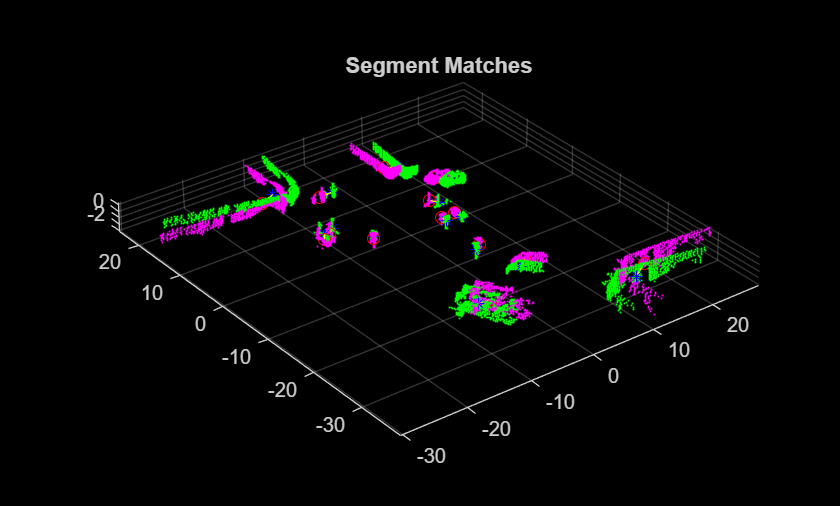Align the segments with the transformation from the geometric verification step using `pccat` and `pctransform`.

```ptCloudSegments1 = pccat(segments1); ptCloudSegments2 = pccat(segments2); tformedPtCloudSegments1 = pctransform(ptCloudSegments1, tform);```

Visualize the aligned segments using `pcshowpair`.

```figure pcshowpair(tformedPtCloudSegments1, ptCloudSegments2) title('Aligned Segments')```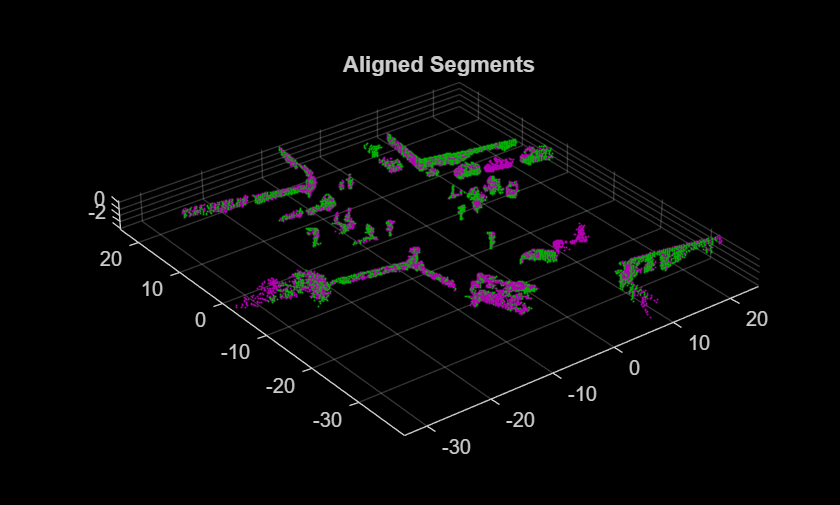### Build Map

The map building procedure consists of the following steps:

1. Preprocess the point cloud

2. Register the point cloud

3. Segment the point cloud and extract features

4. Detect loop closures

#### Preprocess Point Cloud

Preprocess the previous and current point cloud using `helperPreProcessPointCloud`. Downsample the point clouds using `pcdownsample` to improve registration speed and accuracy. To tune the downsample percentage input, find the lowest value that maintains the desired registration accuracy when the vehicle turns.

```currentViewId = 2; prevPtCloud = helperPreProcessPointCloud(ptCloudMap(currentViewId - 1), ... egoRadius, cylinderRadius); ptCloud = helperPreProcessPointCloud(ptCloudMap(currentViewId), ... egoRadius, cylinderRadius); downsamplePercent = 0.5; prevPtCloudFiltered = pcdownsample(prevPtCloud, 'random', downsamplePercent); ptCloudFiltered = pcdownsample(ptCloud, 'random', downsamplePercent);```

#### Register Point Cloud

Register the current point cloud with the previous point cloud to find the relative transformation.

```gridStep = 3; relPose = pcregisterndt(ptCloudFiltered, prevPtCloudFiltered, gridStep);```

Use a `pcviewset` object to track absolute poses and connections between registered point clouds. Create an empty `pcviewset`.

`vSet = pcviewset;`

Initialize the pose of the first point cloud to an identity rigid transformation, and add it to the view set using `addView`.

```initAbsPose = rigid3d; vSet = addView(vSet, currentViewId - 1, initAbsPose);```

Compute the absolute pose of the second point cloud using the relative pose estimated during registration, and add it to the view set.

```absPose = rigid3d(relPose.T * initAbsPose.T); vSet = addView(vSet, currentViewId, absPose);```

Connect the two views using `addConnection`.

`vSet = addConnection(vSet, currentViewId - 1, currentViewId, relPose);`

Transform the current point cloud to align it to the global map.

`ptCloud = pctransform(ptCloud, absPose);`

#### Segment Point Cloud and Extract Features

Segment the previous and current point clouds using `segmentLidarData``.`

```labels1 = segmentLidarData(prevPtCloud, distThreshold, angleThreshold, ... 'NumClusterPoints', minNumPoints); labels2 = segmentLidarData(ptCloud, distThreshold, angleThreshold, ... 'NumClusterPoints', minNumPoints);```

Extract features from the previous and current point cloud segments using `extractEigenFeatures` (Lidar Toolbox).

```[prevFeatures, prevSegments] = extractEigenFeatures(prevPtCloud, labels1); [features, segments] = extractEigenFeatures(ptCloud, labels2);```

Track the segments and features using a `pcmapsegmatch` (Lidar Toolbox) object. Create an empty `pcmapsegmatch` (Lidar Toolbox).

`sMap = pcmapsegmatch;`

Add the views, features, and segments for the previous and current point clouds to the `pcmapsegmatch` (Lidar Toolbox) using `addView` (Lidar Toolbox).

```sMap = addView(sMap, currentViewId - 1, prevFeatures, prevSegments); sMap = addView(sMap, currentViewId, features, segments);```

#### Detect Loop Closures

The estimated poses accumulate drift as more point clouds are added to the map. Detecting loop closures helps correct for the accumulated drift and produce a more accurate map.

Detect loop closures using `findPose` (Lidar Toolbox).

```[absPoseMap, loopClosureViewId] = findPose(sMap, absPose); isLoopClosure = ~isempty(absPoseMap);```

If `findPose` (Lidar Toolbox) detects a loop closure, find the transformation between the current view and the loop closure view and add it to the `pcviewset` object.

Use the absolute pose of the current view without the accumulated drift, `absPoseMap`, and the absolute pose of the loop closure view, `absPoseLoop`, to compute the relative pose between the loop closure poses without the drift.

```if isLoopClosure absPoseLoop = poses(vSet, loopClosureViewId).AbsolutePose; relPoseLoopToCurrent = rigid3d(absPoseMap.T * invert(absPoseLoop).T);```

Add the loop closure relative pose as a connection using `addConnection`.

``` vSet = addConnection(vSet, loopClosureViewId, currentViewId, ... relPoseLoopToCurrent); ```

Correct for the accumulated drift using pose graph optimization. Consider finding more than one loop closure connection before optimizing the poses, since optimizing the pose graph and updating the `pcmapsegmatch` (Lidar Toolbox) object are both computationally intensive.

Save the poses before optimization. The poses are needed to update the segments and centroid locations in the `pcmapsegmatch` (Lidar Toolbox) object.

` prevPoses = vSet.Views.AbsolutePose;`

Create a pose graph from the view set using `createPoseGraph`, and optimize the pose graph using `optimizePoseGraph` (Navigation Toolbox)`.`

``` G = createPoseGraph(vSet); optimG = optimizePoseGraph(G, 'g2o-levenberg-marquardt'); vSet = updateView(vSet, optimG.Nodes);```

Find the transformations from the poses before and after correcting for drift and use them to update the map segments and centroid locations using `updateMap` (Lidar Toolbox).

``` optimizedPoses = vSet.Views.AbsolutePose; relPoseOpt = rigid3d.empty; for k = 1:numel(prevPoses) relPoseOpt(k) = rigid3d(invert(prevPoses(k)).T * ... optimizedPoses(k).T); end sMap = updateMap(sMap, relPoseOpt); end```

To build the map and correct for accumulated drift, apply these steps to the rest of the point cloud scans.

```% Set the random seed for example reproducibility. rng(0) % Update display every 5 scans. figure updateRate = 5; % Initialize variables for registration. prevPtCloud = ptCloudFiltered; prevPose = rigid3d; % Keep track of the loop closures to optimize the poses once enough loop % closures are detected. totalLoopClosures = 0; for i = 3:numel(ptCloudMap) ptCloud = ptCloudMap(i); % Preprocess and register the point cloud. ptCloud = helperPreProcessPointCloud(ptCloud, egoRadius, cylinderRadius); ptCloudFiltered = pcdownsample(ptCloud, 'random', downsamplePercent); relPose = pcregisterndt(ptCloudFiltered, prevPtCloud, gridStep, ... 'InitialTransform', relPose); ptCloud = pctransform(ptCloud, absPose); % Store the current point cloud to register the next point cloud. prevPtCloud = ptCloudFiltered; % Compute the absolute pose of the current point cloud. absPose = rigid3d(relPose.T * absPose.T); % If the vehicle has moved at least 2 meters since last time, continue % with segmentation, feature extraction, and loop closure detection. if norm(absPose.Translation - prevPose.Translation) >= 2 % Segment the point cloud and extract features. labels = segmentLidarData(ptCloud, distThreshold, angleThreshold, ... 'NumClusterPoints', minNumPoints); [features, segments] = extractEigenFeatures(ptCloud, labels); % Keep track of the current view id. currentViewId = currentViewId + 1; % Add the view to the point cloud view set and map representation. vSet = addView(vSet, currentViewId, absPose); vSet = addConnection(vSet, currentViewId-1, currentViewId, ... rigid3d(absPose.T * invert(prevPose).T)); sMap = addView(sMap, currentViewId, features, segments); % Update the view set display. if mod(currentViewId, updateRate) == 0 plot(vSet) drawnow end % Check if there is a loop closure. [absPoseMap, loopClosureViewId] = findPose(sMap, absPose, 'MatchThreshold', 1, ... 'MinNumInliers', 5, 'NumSelectedClusters', 4, 'NumExcludedViews', 150); isLoopClosure = ~isempty(absPoseMap); if isLoopClosure totalLoopClosures = totalLoopClosures + 1; % Find the relative pose between the loop closure poses. absPoseLoop = poses(vSet, loopClosureViewId).AbsolutePose; relPoseLoopToCurrent = rigid3d(absPoseMap.T * invert(absPoseLoop).T); vSet = addConnection(vSet, loopClosureViewId, currentViewId, ... relPoseLoopToCurrent); % Optimize the graph of poses and update the map every time 3 % loop closures are detected. if mod(totalLoopClosures, 3) == 0 prevPoses = vSet.Views.AbsolutePose; % Correct for accumulated drift. G = createPoseGraph(vSet); optimG = optimizePoseGraph(G, 'g2o-levenberg-marquardt'); vSet = updateView(vSet, optimG.Nodes); % Update the map. optimizedPoses = vSet.Views.AbsolutePose; relPoseOpt = rigid3d.empty; for k = 1:numel(prevPoses) relPoseOpt(k) = rigid3d(invert(prevPoses(k)).T * ... optimizedPoses(k).T); end sMap = updateMap(sMap, relPoseOpt); % Update the absolute pose after pose graph optimization. absPose = optimizedPoses(end); end end prevPose = absPose; end end```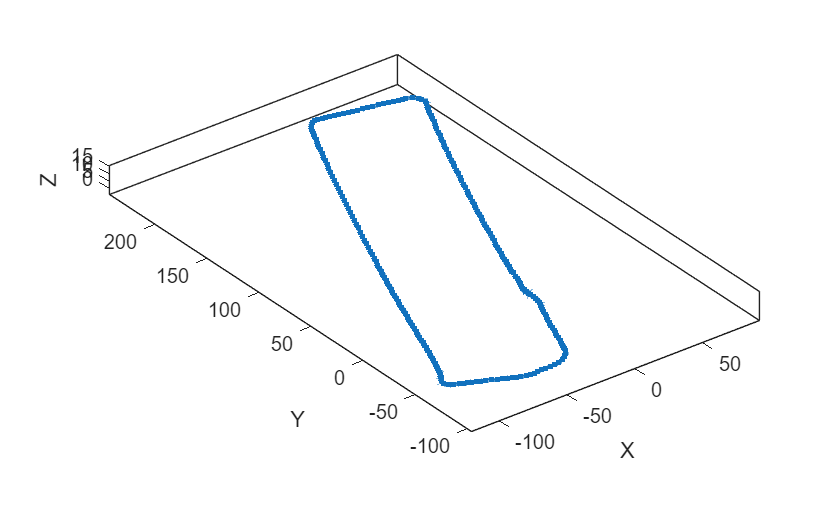```% Visualize the map of segments from the top view. figure show(sMap) view(2) title('Map of Segments')```### Localize Vehicle in Known Map

The preprocessing steps for localization using SegMatch are the same preprocessing steps used for map building. Since the algorithm relies on consistent segmentation, use the same segmentation parameters for best results.

```ptCloud = ptCloudLoc(1); % Preprocess the point cloud. ptCloud = helperPreProcessPointCloud(ptCloud, egoRadius, cylinderRadius); % Segment the point cloud and extract features. labels = segmentLidarData(ptCloud, distThreshold, angleThreshold, ... 'NumClusterPoints', minNumPoints); features = extractEigenFeatures(ptCloud, labels);```

Because there is no position estimate for the vehicle, you must use the extent of the map for initial vehicle localization. Select the extent of the map to localize for the first time using `selectSubmap` (Lidar Toolbox).

`sMap = selectSubmap(sMap, [sMap.XLimits, sMap.YLimits, sMap.ZLimits]);`

Use the `findPose` (Lidar Toolbox) object function of `pcmapsegmatch` (Lidar Toolbox) to localize the vehicle on the prebuilt map.

`absPoseMap = findPose(sMap, features, 'MatchThreshold', 1, 'MinNumInliers', 5);`

Visualize the map, and use `showShape` to visualize the vehicle on the map as a cuboid.

```mapSegments = pccat(sMap.Segments); hAxLoc = pcshow(mapSegments.Location, 'p'); title('Localization on a Prebuilt Map') view(2) poseTranslation = absPoseMap.Translation; quat = quaternion(absPoseMap.Rotation', 'rotmat', 'point'); theta = eulerd(quat, 'ZYX', 'point'); pos = [poseTranslation, 5, 9, 3.5, theta(2), theta(3), theta(1)]; showShape('cuboid', pos, 'Color', 'green', 'Parent', hAxLoc, 'Opacity', 0.8, 'LineWidth', 0.5)```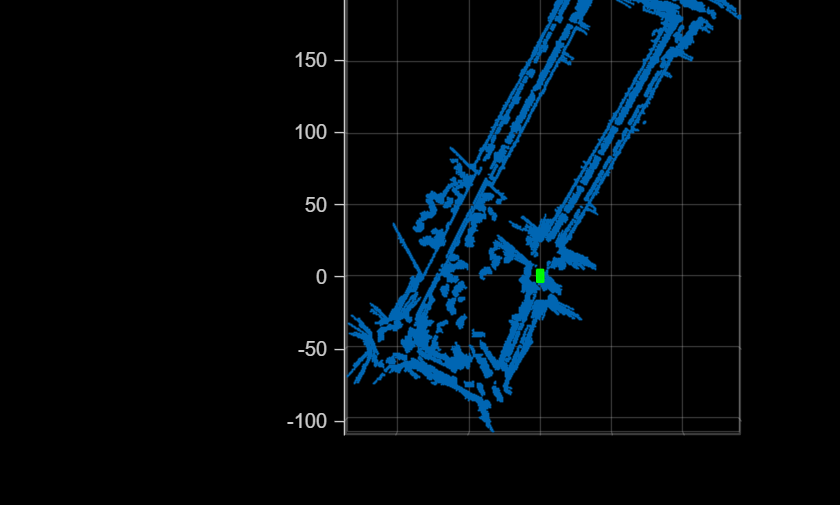To improve localization speed for the rest of the scans, select a submap using `selectSubmap` (Lidar Toolbox).

```submapSize = [65, 65, 200]; sMap = selectSubmap(sMap, poseTranslation, submapSize);```

Continue localizing the vehicle using the rest of the point cloud scans. Use `isInsideSubmap` (Lidar Toolbox) and `selectSubmap` (Lidar Toolbox) to keep the submap updated. If there are not enough segments to localize the vehicle using segment matching, use registration to estimate the pose.

```% Visualize the map. figure('Visible', 'on') hAx = pcshow(mapSegments.Location, 'p'); title('Localization on a Prebuilt Map') % Set parameter to update submap. submapThreshold = 30; % Initialize the poses and previous point cloud for registration. prevPtCloud = ptCloud; relPose = rigid3d; prevAbsPose = rigid3d; % Segment each point cloud and localize by finding segment matches. for n = 2:numel(ptCloudLoc) ptCloud = ptCloudLoc(n); % Preprocess the point cloud. ptCloud = helperPreProcessPointCloud(ptCloud, egoRadius, cylinderRadius); % Segment the point cloud and extract features. labels = segmentLidarData(ptCloud, distThreshold, angleThreshold, ... 'NumClusterPoints', minNumPoints); features = extractEigenFeatures(ptCloud, labels); % Localize the point cloud. absPoseMap = findPose(sMap, features, 'MatchThreshold', 1, 'MinNumInliers', 5); % Do registration when the position cannot be estimated with segment % matching. if isempty(absPoseMap) relPose = pcregisterndt(ptCloud, prevPtCloud, gridStep, ... 'InitialTransform', relPose); absPoseMap = rigid3d(relPose.T * prevAbsPose.T); end % Display position estimate in the map. poseTranslation = absPoseMap.Translation; quat = quaternion(absPoseMap.Rotation', 'rotmat', 'point'); theta = eulerd(quat, 'ZYX', 'point'); pos = [poseTranslation, 5, 9, 3.5, theta(2), theta(3), theta(1)]; showShape('cuboid', pos, 'Color', 'green', 'Parent', hAx, 'Opacity', 0.8, 'LineWidth', 0.5) % Determine if selected submap needs to be updated. [isInside, distToEdge] = isInsideSubmap(sMap, poseTranslation); needSelectSubmap = ~isInside ... % Current pose is outside submap. || any(distToEdge(1:2) < submapThreshold); % Current pose is close to submap edge. % Select a new submap. if needSelectSubmap sMap = selectSubmap(sMap, poseTranslation, submapSize); end prevAbsPose = absPoseMap; prevPtCloud = ptCloud; end```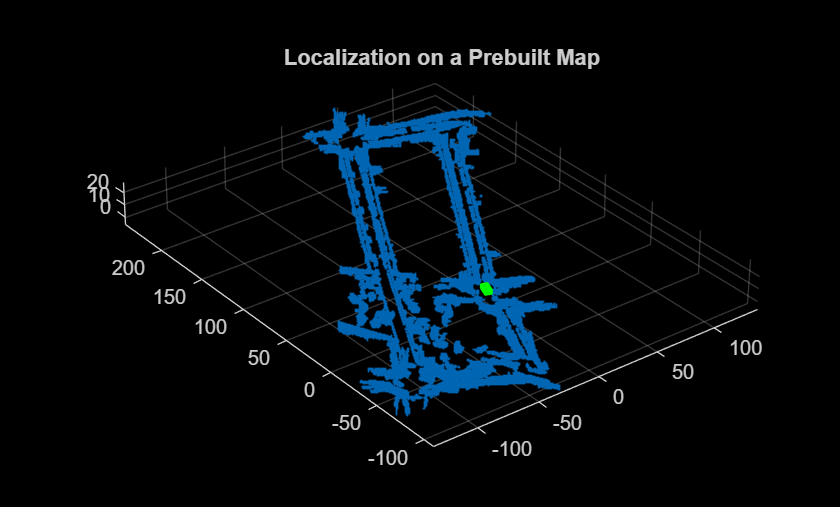### References

 R. Dube, D. Dugas, E. Stumm, J. Nieto, R. Siegwart, and C. Cadena. "SegMatch: Segment Based Place Recognition in 3D Point Clouds." IEEE International Conference on Robotics and Automation (ICRA), 2017.

### Supporting Functions

`helperReadVelodyneSLAMData` reads point clouds from PNG image files from the Velodyne SLAM Dataset.

`helperPreProcessPointCloud` selects a cylindrical neighborhood and removes the ground from a point cloud.

```function ptCloud = helperPreProcessPointCloud(ptCloud, egoRadius, cylinderRadius) % Compute the distance between each point and the origin. dists = hypot(ptCloud.Location(:,:,1), ptCloud.Location(:,:,2)); % Select the points inside the cylinder radius and outside the ego radius. cylinderIdx = dists <= cylinderRadius & dists >= egoRadius; ptCloud = select(ptCloud, cylinderIdx, 'OutputSize', 'full'); % Remove ground. [~, ptCloud] = segmentGroundSMRF(ptCloud, 'ElevationThreshold', 0.05); end```